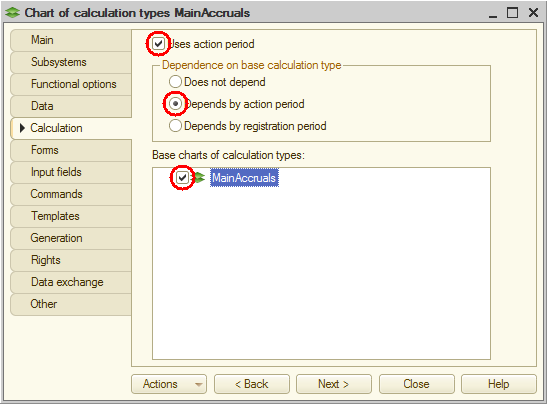# 1C:Enterprise 8.3. Practical Developer’s Guide. Lesson 17 (1:00). Charts of calculation types and calculation registers. Adding a chart of calculation types

## Adding a chart of calculation types

Let us proceed to creating a chart of calculation types named MainAccruals. You will use this chart of calculation types in your configuration.

### In Designer mode

Let us create a chart of calculation types.

1. In Designer, create a ChartOfCalculationTypes object named MainAccruals.
2. In the List presentation field, enter Calculation types.
3. On the Subsystems tab, include the chart of calculation types in the Payroll subsystem.
4. On the Calculation tab, specify that it uses action period and depends on base calculation type by action period.
5. Specify that this chart of calculation types serves as a base chart of calculation types for itself (fig. 17.4).

Since it is the only chart of calculation types in the configuration, it will store all of the calculation types, including the base ones.Fig. 17.4. Specifying the use of action period, dependency by base calculation type, and the base chart of calculation types

Let us specify the predefined calculation types. As with accounting, calculations at Jack of All Trades are rather basic, so you will only create three items.
6. On the Other tab, create the following predefined items (fig. 17.5):
• Absence. Name, code, and description: Absence
• Salary. Name, code, and description: Salary, displacing calculation type: Absence, leading calculation type: Absence
• Bonus. Name, code, and description: Bonus, base calculation type: Salary, leading calculation types: Absence and SalaryFig. 17.5. Predefined calculation types for the MainAccruals chart of calculation types

Now let us proceed to discussing the second object used in the implementation of complex periodic calculations: the Calculation register.